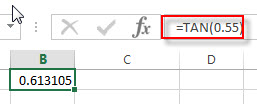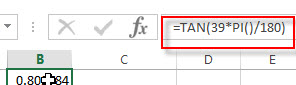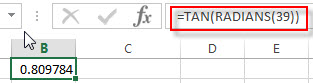## Excel TAN Function

This post will guide you how to use Excel TAN function with syntax and examples in Microsoft excel.

Description

The Excel TAN function returns the tangent of a given angle. So you can use this function to calculate the tangent of an angle in radians. If you want to put an angle to TAN in degrees, you need to multiply the angle by PI()/180, then put the returned result into the TAN function. Or you can use the RADIANS function to combine with the TAN function.

The TAN function is a build-in function in Microsoft Excel and it is categorized as a Math and Trigonometry Function.

The TAN function is available in Excel 2016, Excel 2013, Excel 2010, Excel 2007, Excel 2003, Excel XP, Excel 2000, Excel 2011 for Mac.

Syntax

The syntax of the TAN function is as below:

`=TAN (number)`

Where the TAN function argument is:

• number -This is a required argument. A numeric value that used to calculate the tangent of an angle in radians.

Note:

• If your argument is in degrees, multiply it by PI()/180 or use the RADIANS function to convert it to radians.

Excel TAN Function Examples

The below examples will show you how to use Excel TAN Function to return the tangent of an angle in radians.

1# get the tangent of 0.55 radians, enter the following formula in Cell B1.

`=TAN(0.55)`2# get the tangent of 39 degrees, use the below formula in Cell B2

`=TAN(39*PI()/180)`Or you can use the RADINANS function to convert the angle to radians, then pass the returned value into TAN function to achieve the same result. Type the following formula in Cell B3:

`=TAN(RADIANS(39))`### Related Functions

• Excel TANH function
The Excel TANH function returns the hyperbolic tangent of a given number.The syntax of the TANH function is as below:=TANH (number)…
• Excel SIN function
The Excel TANH function returns the hyperbolic tangent of a given number.The syntax of the TANH function is as below:=TANH (number)…
• Excel ACOS function
The Excel ACOS function returns the arccosine value of a number.The ACOS function is a build-in function in Microsoft Excel and it is categorized as a Math and Trigonometry Function.The syntax of the ACOS function is as below:= ACOS(number)…
• Excel ACOSH function
The Excel ACOSH function returns the inverse hyperbolic cosine of a number.The syntax of the ACOSH function is as below:= ACOSH (number)…
• Excel ASIN function
The Excel ASIN function returns the arcsine value of a number.The syntax of the ASIN function is as below:= ASIN (number)…
• Excel ATAN function
The Excel ATAN function returns the arctangent of a number. So this function can be used to calculates the arctangent of a supplied number. The returned angle is given in radians in the range –pi/2 to pi/2. The syntax of the ATAN function is as below:= ATAN (number)…
• Excel ATAN2 function
The Excel ATAN2 function returns the arctangent, or inverse tangent, of the specified x- and y-coordinates. And you can use the ATAN2 function to calculate the arctangent of a given x and y coordinates. The syntax of the ATAN2 function is as below:= ATAN2 (x_num, y_num)…
• Excel ATANH function
The Excel ATANH function returns the inverse hyperbolic tangent of a given number. The syntax of the ATANH function is as below:= ATANH (number)…
• Excel PI function
The Excel PI function returns the value of mathematical constant PI. The returned value is 3.14159265358979 and ti will accurate to 15 digits.The syntax of the PI function is as below:=PI()…